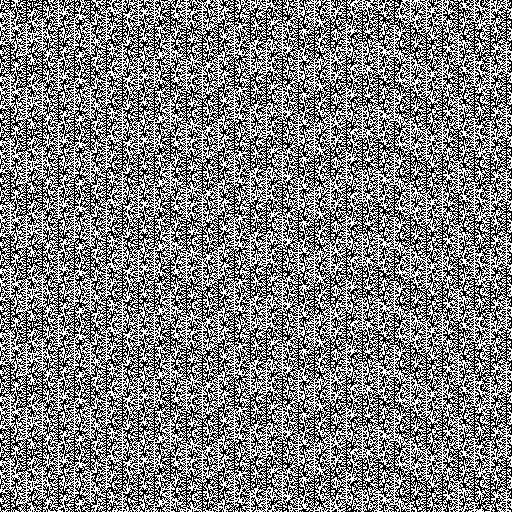## Sunday, 15 June 2014

### Computational Number Theory - Pseudo Random Numbers

Computers are increasingly being used to solve mathematical problems, and are becoming more prominent in solving problems in Number Theory and Graph Theory, as well as, fields of Physics and Biology. However, computers have been used to create seemingly random numbers for either games or security purposes; these seemingly random numbers are called Pseudo-Random. They may seem random but in fact they aren't random at all.

To illustrate the difference between a true random number and a pseudo random number, look a look at the two images I've taken from Bo Allen's blog:True Random NumberPseudo Random Number
The difference is very obvious and thus highlights the key differences between a true random generator and a pseudo random number generator. A pseudo random number generator uses a mathematical algorithm, which is able to produce seemingly random numbers. A true random number generator uses methods which can't be predicted, and therefore are truly random. The randomness of numbers is important for encryption purposes and cryptography.

The true random number generators are hardware based, and most use the physics of Quantum Mechanics and it's probabilistic nature, like the quantization of electromagnetism which lead to discovery of photons and the Photoelectric effect. A pseudo random generator uses a software based mathematical algorithm to generate these random numbers.

A list of random number generators can be found here.